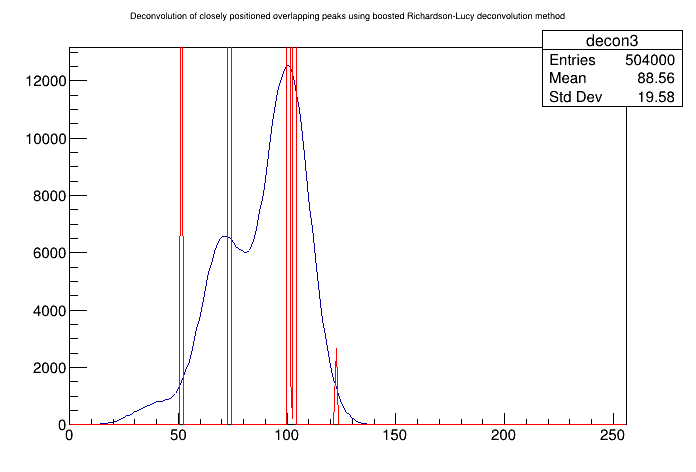# DeconvolutionRL_wide_boost¶

Example to illustrate deconvolution function (class TSpectrum).

Author: Miroslav Morhac, Olivier Couet
This notebook tutorial was automatically generated with ROOTBOOK-izer from the macro found in the ROOT repository on Tuesday, August 09, 2022 at 09:43 AM.

In :
Int_t i;
const Int_t nbins = 256;
Double_t xmin     = 0;
Double_t xmax     = nbins;
Double_t source[nbins];
Double_t response[nbins];
gROOT->ForceStyle();

TString dir  = gROOT->GetTutorialDir();
TString file = dir+"/spectrum/TSpectrum.root";
TFile *f     = new TFile(file.Data());
TH1F* h = (TH1F*) f->Get("decon3");
h->SetTitle("Deconvolution of closely positioned overlapping peaks using boosted Richardson-Lucy deconvolution method");
TH1F* d = (TH1F*) f->Get("decon_response_wide");

for (i = 0; i < nbins; i++) source[i]=h->GetBinContent(i + 1);
for (i = 0; i < nbins; i++) response[i]=d->GetBinContent(i + 1);

h->Draw("L");
TSpectrum *s = new TSpectrum();
s->DeconvolutionRL(source,response,256,200,50,1.2);

for (i = 0; i < nbins; i++) d->SetBinContent(i + 1,source[i]);
d->SetLineColor(kRed);
d->Draw("SAME L");

Info in <TCanvas::MakeDefCanvas>:  created default TCanvas with name c1


Draw all canvases

In :
gROOT->GetListOfCanvases()->Draw()